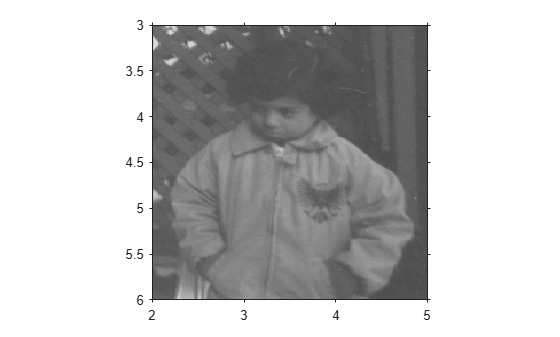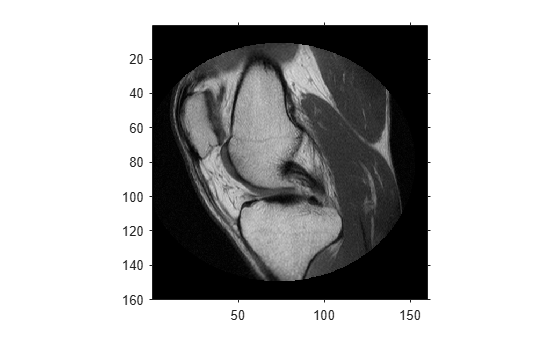Main Content

# imref2d

Reference 2-D image to world coordinates

## Description

An `imref2d` object stores the relationship between the intrinsic coordinates anchored to the rows and columns of a 2-D image and the spatial location of the same row and column locations in a world coordinate system.

The image is sampled regularly in the planar world-x and world-y coordinate system such that intrinsic-x values align with world-x values, and intrinsic-y values align with world-y values. The resolution in each dimension can be different.

## Creation

You can create an `imref2d` object in these ways.

• `affineOutputView` — Store the spatial extent of an image that is warped by a 2-D affine geometric transformation.

• The `imref2d` function described here

### Syntax

``R = imref2d``
``R = imref2d(imageSize)``
``R = imref2d(imageSize,pixelExtentInWorldX,pixelExtentInWorldY)``
``R = imref2d(imageSize,xWorldLimits,yWorldLimits)``

### Description

````R = imref2d` creates an `imref2d` object with default property settings.```
````R = imref2d(imageSize)` sets the optional `ImageSize` property.```

example

````R = imref2d(imageSize,pixelExtentInWorldX,pixelExtentInWorldY)` sets the optional `ImageSize`, `PixelExtentInWorldX`, and `PixelExtentInWorldY` properties.```

example

````R = imref2d(imageSize,xWorldLimits,yWorldLimits)` sets the optional `ImageSize`, `XWorldLimits`, and `YWorldLimits` properties.```

## Properties

expand all

Span of image in the x-dimension in the world coordinate system, specified as a numeric scalar. The `imref2d` object sets this value as `PixelExtentInX * ImageSize(2)`.

Data Types: `double`

Span of image in the y-dimension in the world coordinate system, specified as a numeric scalar. The `imref2d` object sets this value as `PixelExtentInY * ImageSize(1)`.

Data Types: `double`

Number of elements in each spatial dimension, specified as a 2-element positive row vector. `ImageSize` is the same form as that returned by the `size` function.

Data Types: `double`

Size of a single pixel in the x-dimension measured in the world coordinate system, specified as a positive number.

Data Types: `double`

Size of a single pixel in the y-dimension measured in the world coordinate system, specified as a positive number.

Data Types: `double`

Limits of image in world x-dimension, specified as a 2-element row numeric vector `[xMin xMax]`.

Data Types: `double`

Limits of image in world y-dimension, specified as a 2-element numeric row vector `[yMin yMax]`.

Data Types: `double`

Limits of image in intrinsic units in the x-dimension, specified as a 2-element row vector `[xMin xMax]`. For an m-by-n image (or an m-by-n-by-p image), `XIntrinsicLimits` equals ```[0.5, n+0.5]```.

Data Types: `double`

Limits of image in intrinsic units in the y-dimension, specified as a 2-element row vector `[yMin yMax]`. For an m-by-n image (or an m-by-n-by-p image), `YIntrinsicLimits` equals ```[0.5, m+0.5]```.

Data Types: `double`

## Object Functions

 `contains` Determine if image contains points in world coordinate system `intrinsicToWorld` Convert from intrinsic to world coordinates `sizesMatch` Determine if object and image are size-compatible `worldToIntrinsic` Convert from world to intrinsic coordinates `worldToSubscript` Convert world coordinates to row and column subscripts

## Examples

collapse all

Read a 2-D grayscale image into the workspace.

`A = imread('pout.tif');`

Create an `imref2d` object, specifying the size and world limits of the image associated with the object.

```xWorldLimits = [2 5]; yWorldLimits = [3 6]; RA = imref2d(size(A),xWorldLimits,yWorldLimits)```
```RA = imref2d with properties: XWorldLimits: [2 5] YWorldLimits: [3 6] ImageSize: [291 240] PixelExtentInWorldX: 0.0125 PixelExtentInWorldY: 0.0103 ImageExtentInWorldX: 3 ImageExtentInWorldY: 3 XIntrinsicLimits: [0.5000 240.5000] YIntrinsicLimits: [0.5000 291.5000] ```

Display the image, specifying the spatial referencing object. The axes coordinates reflect the world coordinates.

```figure imshow(A,RA);```Read a 2-D grayscale image into the workspace.

```m = dicominfo('knee1.dcm'); A = dicomread(m);```

Create an `imref2d` object, specifying the size and the resolution of the pixels. The DICOM file contains a metadata field `PixelSpacing` that specifies the image resolution in each dimension in millimeters per pixel.

`RA = imref2d(size(A),m.PixelSpacing(2),m.PixelSpacing(1))`
```RA = imref2d with properties: XWorldLimits: [0.1562 160.1562] YWorldLimits: [0.1562 160.1562] ImageSize: [512 512] PixelExtentInWorldX: 0.3125 PixelExtentInWorldY: 0.3125 ImageExtentInWorldX: 160 ImageExtentInWorldY: 160 XIntrinsicLimits: [0.5000 512.5000] YIntrinsicLimits: [0.5000 512.5000] ```

Display the image, specifying the spatial referencing object. The axes coordinates reflect the world coordinates.

```figure imshow(A,RA,'DisplayRange',[0 512])```Compare the width of the image in world coordinates and intrinsic coordinates. This image width in intrinsic coordinates, with units of pixels, is:

`RA.ImageSize(1)`
```ans = 512 ```

The image width in world coordinates, with units of millimeters, is:

`RA.ImageExtentInWorldX`
```ans = 160 ```

expand all

## Tips

• You can create an `imref2d` object for an RGB image. If you create the object specifying the `ImageSize` property as a three-element vector (such as that returned by the `size` function), only the first two elements are used to set `ImageSize`.

## See Also

Introduced in R2013a

Download ebook